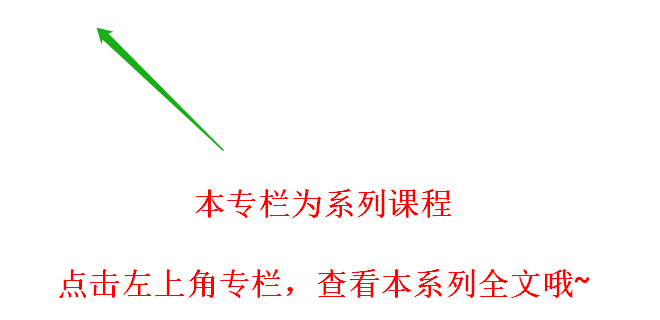# 神经网络理论基础及Python实现

+关注继续查看……4.1、算法详细介绍

1、由输入层向前传送：Ij为当前层单元值，Oi为上一层的单元值，wij为两层之间，连接两个单元值的权重值，sitaj为每一层的偏向值。我们要对每一层的输出进行非线性的转换，示意图如下：2、根据误差反向传送 对于输出层：其中Tk是真实值，Ok是预测值3、终止条件

4、非线性转化函数

(1)tanh(x)函数：

• tanh(x)=sinh(x)/cosh(x)
• sinh(x)=(exp(x)-exp(-x))/2
• cosh(x)=(exp(x)+exp(-x))/2

(2)逻辑函数，本文上面用的就是逻辑函数

1. import numpy as np

1. def tanh(x):
2.     return np.tanh(x)
3. def tanh_deriv(x):
4.     return 1.0 - np.tanh(x)*np.tanh(x)
5. def logistic(x):
6.     return 1/(1 + np.exp(-x))
7. def logistic_derivative(x):
8.     return logistic(x)*(1-logistic(x))

1. class NeuralNetwork:
2.     def __init__(self, layers, activation='tanh'):
3.         ""
4.         :param layers: A list containing the number of units in each layer.
5.         Should be at least two values
6.         :param activation: The activation function to be used. Can be
7.         "logistic" or "tanh"
8.         ""
9.         if activation == 'logistic'
10.             self.activation = logistic
11.             self.activation_deriv = logistic_derivative
12.         elif activation == 'tanh'
13.             self.activation = tanh
14.             self.activation_deriv = tanh_deriv
15.
16.         self.weights = []
17.         for i in range(1, len(layers) - 1):
18.             self.weights.append((2*np.random.random((layers[i - 1] + 1, layers[i] + 1))-1)*0.25)
19.             self.weights.append((2*np.random.random((layers[i] + 1, layers[i + 1]))-1)*0.25)

1. def fit(self, X, y, learning_rate=0.2, epochs=10000):
2.        X = np.atleast_2d(X)
3.        temp = np.ones([X.shape, X.shape+1])
4.        temp[:, 0:-1] = X
5.        X = temp
6.        y = np.array(y)
7.
8.        for k in range(epochs):
9.            i = np.random.randint(X.shape)
10.            a = [X[i]]
11.
12.            for l in range(len(self.weights)):
13.                a.append(self.activation(np.dot(a[l], self.weights[l])))
14.            error = y[i] - a[-1]
15.            deltas = [error * self.activation_deriv(a[-1])]
16.
17.            for l in range(len(a) - 2, 0, -1):
18.                deltas.append(deltas[-1].dot(self.weights[l].T)*self.activation_deriv(a[l]))
19.            deltas.reverse()
20.
21.            for i in range(len(self.weights)):
22.                layer = np.atleast_2d(a[i])
23.                delta = np.atleast_2d(deltas[i])
24.                self.weights[i] += learning_rate * layer.T.dot(delta)

1. def predict(self, x):
2.        x = np.array(x)
3.        temp = np.ones(x.shape+1)
4.        temp[0:-1] = x
5.        a = temp
6.        for l in range(0, len(self.weights)):
7.            a = self.activation(np.dot(a, self.weights[l]))
8.        return a

1. from BP import NeuralNetwork
2. import numpy as np
3.
4. nn = NeuralNetwork([2,2,1], 'tanh'
5. x = np.array([[0,0], [0,1], [1,0], [1,1]])
6. y = np.array([1,0,0,1])
7. nn.fit(x,y,0.1,10000)
8. for i in [[0,0], [0,1], [1,0], [1,1]]:
9.     print(i, nn.predict(i))

1. ([0, 0], array([ 0.99738862]))
2. ([0, 1], array([ 0.00091329]))
3. ([1, 0], array([ 0.00086846]))
4. ([1, 1], array([ 0.99751259]))20 0【Pytorch神经网络基础理论篇】 07 线性回归 + 基础优化算法

14 0【Pytorch神经网络基础理论篇】 04 线性代数

19 0【Pytorch神经网络基础理论篇】 01 从零开始介绍深度学习算法和代码实现

23 030 0【机器学习】线性分类——感知机算法（理论+图解+公式推导）
【机器学习】线性分类——感知机算法（理论+图解+公式推导）
42 0【机器学习】集成学习（Boosting）——XGBoost算法（理论+图解+公式推导）
【机器学习】集成学习（Boosting）——XGBoost算法（理论+图解+公式推导）
98 0【机器学习】决策树——ID3和C4.5（理论+图解+公式推导）
【机器学习】决策树——ID3和C4.5（理论+图解+公式推导）
45 0【机器学习】线性分类——朴素贝叶斯分类器NBC（理论+图解+公式推导）
【机器学习】线性分类——朴素贝叶斯分类器NBC（理论+图解+公式推导）
35 0

【Python入门】Python数据处理编程基础3426